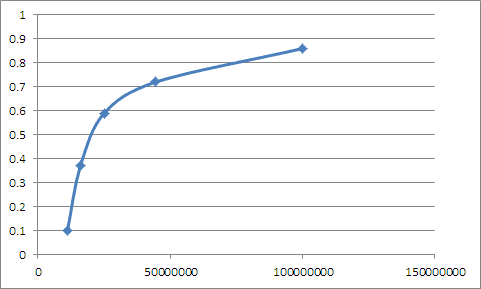# Linear fit for data (resistance of a wire)

## Homework Statement

The resistance for a wire with cross-sectional radius r is given by R = k/(r^2). Convert this equation to a straight line for which k can be determined from the slope.

## Homework Equations

Equation of a line is:
y = mx + c

## The Attempt at a Solution

R = k/(r^2)
ln(R) = ln(k/(r^2))
ln(R) = ln(k) - ln(r^2)
ln(R) = ln(k) - 2*ln(r)

I'm not sure how to linearize this equation, this is where I get stuck. ln(R) would represent y on the graph. k ought to equal the slope, but I can't see how to do that.

What you have done is a perfectly good analysis but k is given by the intercept (lnk) on the graph.
The slope of the ln-ln graph is -2. This is the value of ln-ln graphs.....enables you to determine the power law of a set of data.
Since R =k/r^2 a graph of R against 1/(r^2) will be a straight line with gradient k

The problem is that if I plot given values of R on the y-axis = R and given values of r on the x-axis = (1/(r^2)), my graph looks like this:Edit: This is my dataset
R r
0.81 0.0001
0.73 0.00015
0.60 0.0002
0.38 0.00025
0.1 0.0003

Last edited:
sophiecentaur
Gold Member
Why not plot a graph of R against 1/r2?
That should give a straight line y=kx which should pass through the origin as when r = 0, so should R. The c would be zero.

It is handy to get used to the idea of choosing what to plot against what in order to get a straight line graph. I remember needing quite a few examples before it became 'obvious' what to do.

That graph I showed is R against 1/(r^2). My problem is that it is very curved, so I don't have a best-fit line.

If I use some known value of k to produce my own table of r and R from R = k/(r^2), I do get a linear fit, so I guess I'm missing something involving the dataset.

I have a similar small question, how would I convert this to a straight line such that a and b can be found? My known values are x and y.
y = a*(1 + b*(x^2))

y = a + a*b*(x^2)
ln(y) = ln(a) + ln(a*b*(x^2))
ln(y) = ln(a) + ln(a) + ln(b*(x^2)
ln(y) = 2ln(a) + ln(b*(x^2))
I can see that the intercept is 2ln(a), but I'm not sure how I would read a slope from ln(b*(x^2))

I used your numbers and got something very similar! Where did you get the data, was it from measurements you made?

The data was given to me as part of the question by my instructor.

Could also you please also take a look at my second question in post #6?

What was post #6? I am not certain how to find it

Got it (a bit on the slow side!!)
You can't do this:
y = a + a*b*(x^2)
ln(y) = ln(a) + ln(a*b*(x^2))
You cannot add the ln of 2 numbers added together.When you add ln it means you are dealing with multiplication. You did this right in your post #1
For this equation I would leave it as:
y = a + abx^2
Try plotting y against x^2..... what will be the gradient and what will be the intercept?

When I added, I was thinking of ln(x) + ln(x) = ln(x*x) = 2*ln(x)

D'oh... if the intercept is a and gradient is ab, then I can find b as gradient/a, thanks.you have got it
Cheers

sophiecentaur